# Activation functions and weight initialization in deep learning

Yes, let me begin the initial step of yours in deep learning by teaching you the two basic and important concepts in deep learning i.e.,Activation functions and weight initialization in deep learning.

# Activation functions

Intro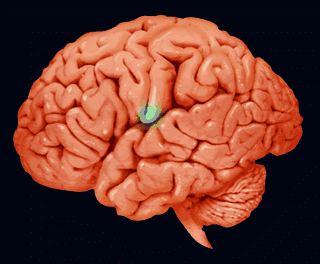Image link : http://bit.ly/2Wy2wQu

For everything there is biological inspiration. Activation functions and neural networks are one of the beautiful ideas inspired from humans. When we feed lots of information to our brain, it tries hard to understand and classify the information between useful and not so useful information. In the same way, we need similar mechanism to classify the incoming information as useful and not useful in case of neural networks. Only some part of information is much useful and rest may be some noise. Network tries to learn the useful information. For that, we need activation functions. Activation function helps the network in doing segregation. In simpler words, activation function tries to build the wall between useful and less useful information.

Let me introduce you to some terminologies, inorder to simplify understanding .

. Neural networks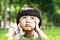Image link : http://bit.ly/2DRZOy8

Let me give a simple example and later i will connect the dots with the theory. Suppose, we are teaching an 8 year old kid to perform addition of two numbers. First of all, he will receive the information about how to perform addition from the instructor. He now tries to learn from the information given and finally , he performs addition. Here, the kid can be thought as neuron, it tries to learn from the input given and finally from the neuron we will get output.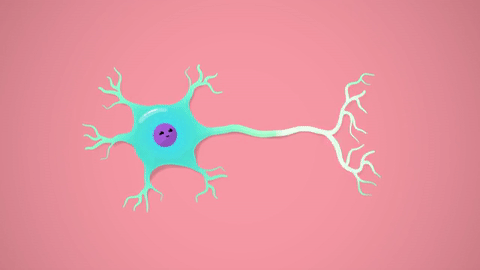Image link : http://bit.ly/2GmJdnN

In biological perspective, this ideal is similar to human brain . Brain receives the stimulus from outside world, does processing on the input and then generates the output. As the task gets more complex, multiple neurons form a complex network passing information among themselves.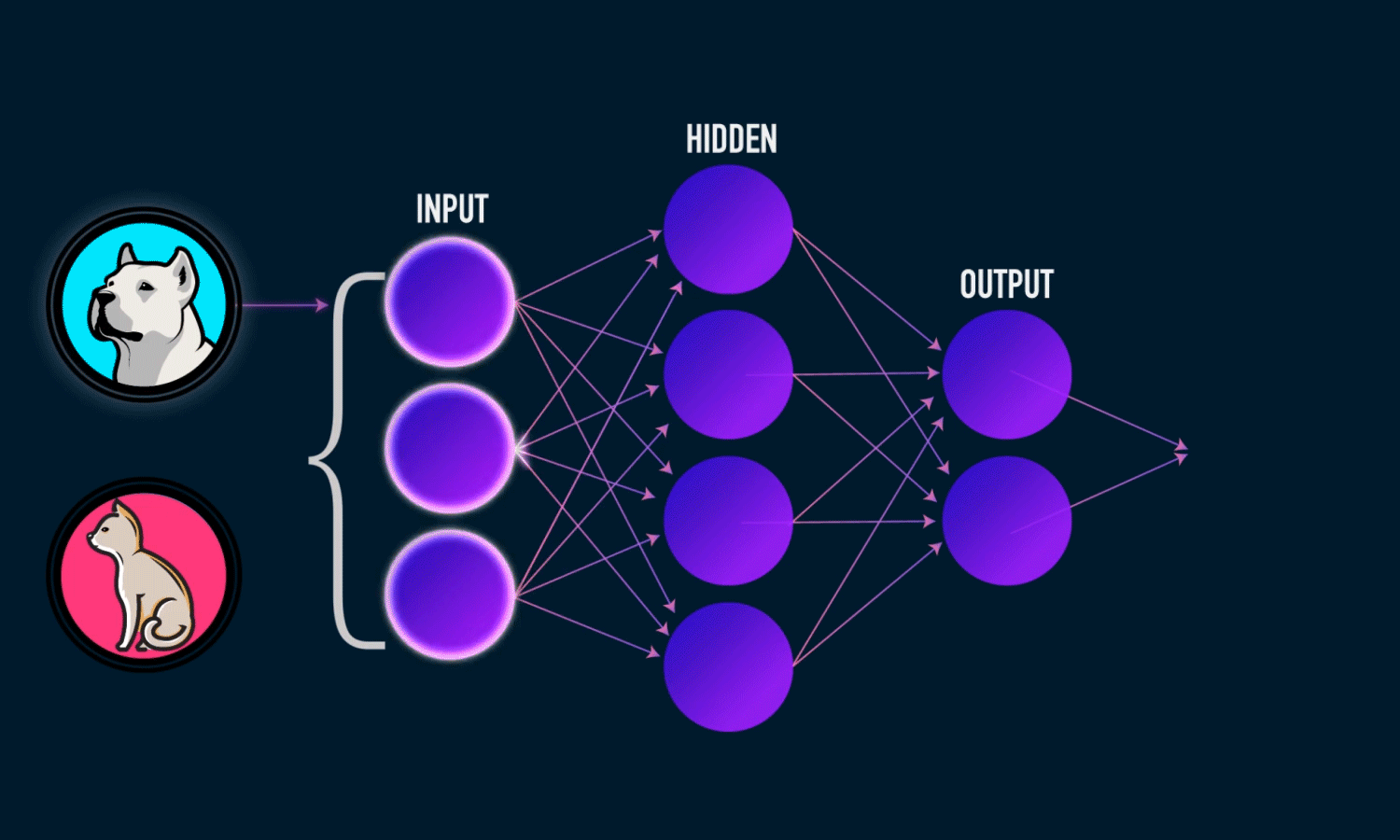Image link : http://bit.ly/2DRuKOW

The blue circles are the neurons. Each neuron has weight,bias and activation function. Input is fed to the input layer. The neuron then performs a linear transformation on the input by the weights and biases. The non linear transformation is done by the activation function.The information moves from input layer to hidden layer. Hidden layer would do the processing and gives output. This mechanism is forward propagation.

What if the output generated is far away from the expected value ?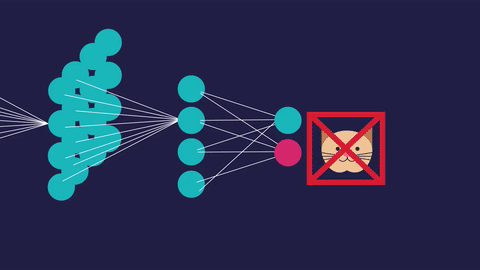Image link : http://bit.ly/2G9hM1u

In neural network, we would update the weights and biases of the neurons on the biases of error. This process is known as back propagation. Once the entire data has gone through this process, final weights and biases are used for predictions.

.Vanishing gradients

Generally, adding more number of hidden layers in the network will allows it to learn more complex functions, thus it performs well.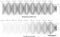Image link : http://bit.ly/2WxRUkC

But, here comes the problem, when we do back propagation i.e., calculating and updating the weights in backward direction,the gradients tends to get smaller and smaller as we keep on moving backwards in the network. This means the weights of the neurons in the earlier layers learn very slowly or sometimes they won’t change at all .But earlier layers in the network are much important because they are responsible for detecting simple patterns. If the earlier layers give inappropriate results,then how can we expect our model to perform well in later layers. This problem is called vanishing gradient problem.

. Exploding gradients

We know that, when we have more number of hidden layers, our model tends to perform well. When we do back propagation, if the gradients become larger and larger, then the weights of the neurons in the earlier stages change much. We know that the earlier layers are much important. Because of this larger weights, the neurons in the earlier layers will give inappropriate results. This problem is called exploding gradients problem.

Now, let us dive deep into core concept of activation functions.

What is an activation function ?

An activation function is a non-linear function applied by the neuron to introduce non-linear properties in the network.”

Let me explain in detail. There are two types of functions i.e., linear and non-linear functions.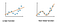Image link : http://bit.ly/2t5j7O3

.Linear function

If the change in the first variable corresponds to a constant change in the second variable, then we call it as linear function.

.Non-linear function

If the change in the first variable doesn’t necessarily correspond with a constant change in the second variable, then we call it as non-linear function.

Why we use activation functions ?

In simple case of any neural network, we multiply weights with the input, add bias and apply an activation function and pass the output to the next layer and we do back propagation to update the weights.

Neural networks are functions approximators. The main goal of any neural network is to learn complex non-linear functions. If we don’t apply any non-linearity in our neural network, we are just trying to separate the classes using a linear hyper plane. As we know, nothing is linear in this real world.

If we perform simple linear operation i.e., multiply the input by weight,add a bias term and sum them across all the inputs arriving to the neuron. In some cases, the output of the above values is very large. When, this output is fed to the further more layers, the values become even more larger , making things computationally uncontrollable. This is where the activation function plays a major role i.e., activation function squashes the input real number to a fixed interval i.e., (between -1 and 1) or (between 0 and 1) .

Let us discuss about the different activation functions and their problems

## .Sigmoid

Sigmoid is a smooth function and is continuously differentiable. This is a non-linear function and it looks like S- shape.Main reason to use sigmoid function is, its value exists between 0 and 1. Therefore, it is especially used for models where we have to predict the probability as an output. Since probability of anything exists between the range 0 and 1, sigmoid is right choice.

As we know, sigmoid function squashes the output values between 0 and 1. In mathematical representation, a large negative number passed through the sigmoid function becomes 0 and and a large positive number becomes 1.

Graph of sigmoid function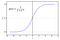Image link : http://bit.ly/2D6UuFm

. The values of sigmoid function is high between the values of -3 and 3 but gets flatter in other regions.

Graph of sigmoid derivative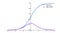Image link : http://bit.ly/2RB5MqD

. Sigmoid function is easily differentiable and the values are dependent on x values. This means that during back propagation, we can easily use sigmoid function to update weights.

Gradient values of sigmoid

Gradient values of sigmoid range between 0 and 0.25 .

Equation of sigmoid function and its derivatives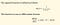Image link : http://bit.ly/2S7Q8bp

Code for sigmoid function in python

`def sigmoid(z): return 1 / (1 + np.exp(-z))`

When we write code for sigmoid, we can use this code for both forward propagation and to compute derivatives .

Problems with sigmoid function

• Values obtained from sigmoid function are not zero centered.
• We can easily face the issue of vanishing gradients and exploding gradients.

Let me explain you how sigmoid function face the problem of vanishing gradients and exploding gradients

Vanishing gradients problem for sigmoid function

Exploding gradients problem for sigmoid

## .Tanh

Tanh function is similar to sigmoid function. Working of tanh function is also similar to the sigmoid function but it is symmetric over the origin . It is continuous and differentiable at all points. It basically takes a real valued number and squashes values to between -1 and 1. Similar to sigmoid neuron, it saturates at large positive and negative values. The output of tanh is always zero centered. Tanh functions are preferred in hidden layers over sigmoid.

Graph of Tanh function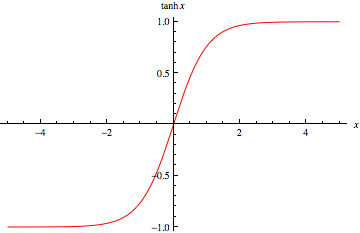Image link : http://bit.ly/2Bhp3rG

. Tanh function takes the real valued function and outputs the values between -1 and 1.

Graph of derivative of tanh function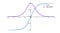Image link : http://bit.ly/2t3WNnW

.The derivative of the tanh function is steeper as compared to the sigmoid function.

. Graph of the tanh function is flat and the gradients are very low.

Equation of tanh function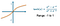Image link : http://bit.ly/2RyLYUZ

Code for tanh function in python

`def tanh(z): return np.tanh(z)`

Gradient values of tanh

Gradient values of tanh range between 0 and 1.

Problems with tanh function

• We can easily face the issue of vanishing gradients and exploding gradients in tanh function also.

Let me explain you how Tanh function face the problem of vanishing gradients and exploding gradients

Vanishing gradients problem for tanh function

Exploding gradients problem for tanh function

## .ReLU

ReLU means Rectified Linear Unit. This is the mostly used activation unit in deep learning. R(x)=max(0,x) i.e., if x<0, R(x)=0 and if x≥0,R(x)=x. It also accelerates the convergence of stochastic gradient descent as compared to sigmoid or tanh activation functions. Main advantage of using the ReLU function is, it does not activate all the neurons at the same time i.e., if the input is negative, it will convert to zero and the neuron does not get activated. This means only a few neurons are activated making the network easy for computation. It also avoids and rectifies vanishing gradient descent problem. Almost all deep learning models use ReLU activation function nowadays.

How do you say ReLU is a non-linear function ?

Linear functions are straight line functions. But, ReLU is not a straight line function because it has bend a at value zero. Hence,we can say that ReLU is a non-linear function. Please have a look at graph of ReLU function.

Graph for ReLU function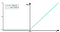Image link : http://bit.ly/2UCQ9kv

. If the value of x is greater than or equal to zero then the we take ReLU(x)=x.

. If the value of x is less than zero then we take ReLU(x)=0.

Graph for derivative of ReLU function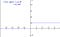Image link : http://bit.ly/2t4jCbp

. If the value of x is greater than zero, then the derivative of the ReLU(x) i.e., ReLU’(x)=1.

. If the value of x is less than zero, then the derivative of the ReLU(x) i.e., ReLU’(x)=0.

Problem with ReLU function

.Dead neurons -

If the units are not activated initially, then during back propagation, zero gradients flow through them. Hence, neurons that already died won’t respond to the variation in the output and the weights will never get updated during back propagation. This problem is called as dead neurons problem.

Equation of ReLU function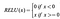Image link : http://bit.ly/2HLdEpQ

Equation of derivative of ReLU function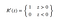Image link : http://bit.ly/2MSnIMU

Code for ReLU activation in python

`def relu(z): return z * (z > 0)`

## . Leaky ReLU

Leaky ReLU is an improved version of ReLU function. We know that in ReLU, the gradient is 0, for x<0. Here in Leaky ReLU, instead of defining the ReLU function as 0, for x<0, we define it as a multiple of small linear component of x i.e., 0.01x (Generally we take linear component as 0.01). The main advantage in Leaky ReLU is, we are just replacing horizontal line on x-axis to non-zero and non horizontal line. We are doing this to remove zero gradient. So, by removing the zero gradients, we won’t face any issue of dead neurons.

Graph of Leaky ReLU function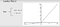Image link : http://bit.ly/2Spy9fU

. If the value of x is greater than zero, then the Leaky ReLU(x)=x.

.If the value of x is less than zero, then the Leaky ReLU(x)=0.01*x.

Graph of derivative of Leaky ReLU function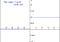Image link : http://bit.ly/2BePOgn

. If the value of x >0, then the derivative of Leaky ReLU(x) i.e., Leaky ReLU’(x)=1.

. If the value of x <0, then the derivative of Leaky ReLU(x) i.e., Leaky ReLU’(x)=0.01.

Equation of Leaky ReLU and derivative of Leaky ReLU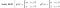Image link : http://bit.ly/2RC1ejU

Here alpha is the small linear component of x . Typically we take alpha value as 0.01 .

Code of Leaky ReLU in python

`def leaky_relu(z): return np.maximum(0.01 * z, z)`

Let me keep all the graphs at one place. So, that you can easily understand the difference between them.

Graphs of activation functions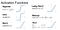Image link : http://bit.ly/2DTfMrH

Graphs of derivative of activation functions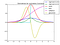Image link : http://bit.ly/2Tq3ix3

Some complex terms like Maxout and ELU are not covered.

Let me keep all the activation function equations and their derivatives at one place, So that you can easily catch up and rewind them easily.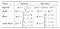Image link : http://bit.ly/2DRAf07

How to choose the right activation function ?

Depending upon the properties of the given problem, we might be able to make a choice and can make a faster convergence of the network.

• Sigmoid functions work better in case of classifiers.
• ReLU is general activation function and can be used in most cases.
• If we encounter dead neurons in our network, then Leaky ReLU is the best choice.

As a rule of thumb, we can begin with ReLU activation function and we can move to other activation functions, if ReLU does not perform well in our network.

# Weight initialization

IntroImage link : http://bit.ly/2WI4mOG

Building a neural network is a tidous task and upon that tuning it to get better result is more challenging. The first challenging task that comes into consideration while building a neural network is initialization of weights, if the weights are initialized correctly, then optimization will be achieved in least time, Otherwise converging to minima is impossible.

Let us have an overview of whole neural network process and the reason why initialization of weights impact’s our model

• Neural network process

Whole neural network process can be explained in 4 steps :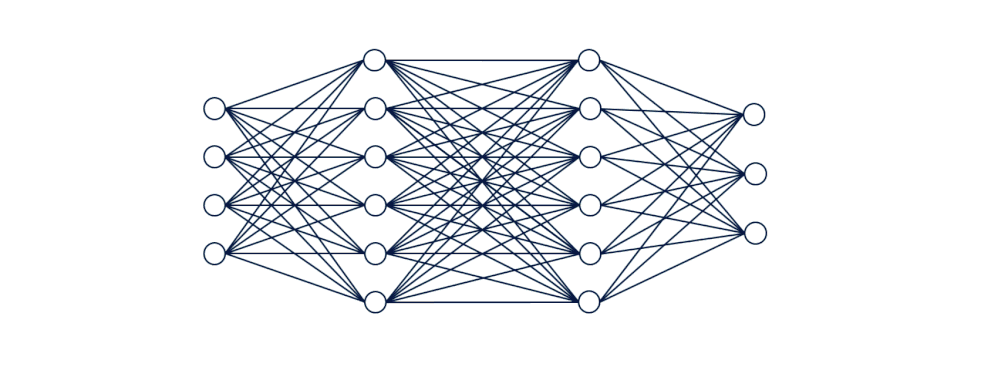Image link : http://bit.ly/2Dcvw7M

1. Initialize weights and biases.

2. Forward propagation : With the weights,inputs and bias term, we multiply the weights with the input and we will add the bias term and then we will perform summation and then we pass this to activation function. This process continues to all the neurons and finally we will get predicted y_hat. This process is called forward propagation.

3. Compute loss function : Difference between the predicted y_hat and the actual y is called loss term. It captures how far our predictions are from the actual target. Our main objective is to minimize the loss function.

4. Back propagation : Here, we compute the gradients and update the weights with respect to loss function . We perform the updation of weights until we get minimum loss.

Steps 2–4 are repeated for n-iterations till we get minimized loss.

By seeing the above neural network process, we can easily say that, steps 2,3 and 4 functionality is same for any network i.e., we do same operations until we converge to minimum loss, only the big difference for faster convergence to minima in any neural network is right initialization of weights .

Now, let us see the different types of initialization of weights. Before going into the topic ,let me introduce you to some terminologies

. Fan-in :

Fan-in is the number of inputs that are entering into the neuron.

. Fan-out :

Fan-out is number of outputs that are going from the neuron.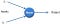Image link : http://bit.ly/2RCK9GD

. There are two inputs that are entering into the neuron. Hence, fan-in=2.

.One output is going away from neuron. Hence, fan-out=1 .

. Uniform distribution :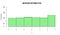Image link : http://bit.ly/2t5jqZm

. Uniform distribution is a type of probability distribution in which all outcomes are equally likely i.e., each variable has the same probability that it will be outcome.

. Normal distribution :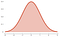Image link : http://bit.ly/2t5iIva

. Normal distribution is a probability distribution that is symmetric about the mean, showing that data near the mean are more frequent in occurrence than the data far from the mean.

Now, let us dive deep into the different initialization techniques. From here, we will go in a practical aspect i.e., Let us take MNIST dataset, and we will initialize the weights with different initialization techniques and let us see what’s happening with output.

Overview of MNIST dataset

MNIST dataset is one of the most common datasets used for image classification. This dataset contains hand written number images and we have to classify them into any one of the 10 classes(i.e., 0 - 9).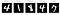Image link : http://bit.ly/2Sm6GvG

For simplicity, we will consider only a 2 layer neural network i.e., 1st hidden layer with 128 neurons , 2nd hidden layer with 64 neurons and we will a softmax classifier to classify the outputs. Here, we will use ReLU as an activation unit. Ok , Lets get started.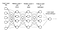Image link : http://bit.ly/2ScKp43

# . Initializing all weights to zero

Theory

Weights are initialized with zero. Then, all the neurons of all the layers performs same calculation, giving same output. The derivative with respect to loss function is same for every weight. The model won’t learn anything. The weight’s won’t get update at all. Here, we are facing vanishing gradients problem.

Code for initializing all weights to zero

`model = Sequential()model.add(Dense(128, activation='relu', input_shape=(input_dim,), kernel_initializer='zeros'))model.add(Dense(64, activation='relu', kernel_initializer='zeros'))model.add(Dense(output_dim, activation='softmax'))`

Output for initializing all weights to zero in MNIST dataset.

`Epoch 1/5 60000/60000 [==============================] - 3s 55us/step - loss: 2.3016 - acc: 0.1119 - val_loss: 2.3011 - val_acc: 0.1135Epoch 2/5 60000/60000 [==============================] - 3s 47us/step - loss: 2.3013 - acc: 0.1124 - val_loss: 2.3010 - val_acc: 0.1135 Epoch 3/5 60000/60000 [==============================] - 3s 46us/step - loss: 2.3013 - acc: 0.1124 - val_loss: 2.3010 - val_acc: 0.1135 Epoch 4/5 60000/60000 [==============================] - 3s 47us/step - loss: 2.3013 - acc: 0.1124 - val_loss: 2.3010 - val_acc: 0.1135 Epoch 5/5 60000/60000 [==============================] - 3s 46us/step - loss: 2.3013 - acc: 0.1124 - val_loss: 2.3010 - val_acc: 0.1135`

Plot for output values for initializing all weights to zero

Analysis of output

Here, the train loss and test loss are not changing . Hence, we can easily conclude that no change in weights of neuron. From this, we can conclude that, our model is effected with vanishing gradients problem.

# . Random initialization of weights

Theory

Instead of initializing all the weights to zeros, here we are initializing all the values to random values. Random initialization is better than zero initialization of weights. But, in random initialization we have chance of facing two issues i.e., vanishing gradients and exploding gradients. If the weights are initialized very high, then we will be facing issue of exploding gradients. If the weights are initialized very low, then we will be facing issue of vanishing gradients.

Code for random initialization of weights

`model = Sequential()model.add(Dense(128, activation='relu', input_shape=(input_dim,), kernel_initializer='random_uniform'))model.add(Dense(64, activation='relu', kernel_initializer='random_uniform'))model.add(Dense(output_dim, activation='softmax'))`

Output for initialization of all weights to random in MNIST dataset

`Epoch 1/5 60000/60000 [==============================] - 3s 55us/step - loss: 0.3929 - acc: 0.8887 - val_loss: 0.1889 - val_acc: 0.9432 Epoch 2/5 60000/60000 [==============================] - 3s 45us/step - loss: 0.1570 - acc: 0.9534 - val_loss: 0.1247 - val_acc: 0.9622 Epoch 3/5 60000/60000 [==============================] - 3s 53us/step - loss: 0.1069 - acc: 0.9685 - val_loss: 0.0994 - val_acc: 0.9705 Epoch 4/5 60000/60000 [==============================] - 3s 54us/step - loss: 0.0810 - acc: 0.9761 - val_loss: 0.0986 - val_acc: 0.9710 Epoch 5/5 60000/60000 [==============================] - 3s 54us/step - loss: 0.0629 - acc: 0.9804 - val_loss: 0.0877 - val_acc: 0.9755`

Plot for outputs that their weights are randomly initialized

Analysis of output

Here, the train loss and the test loss are changing much i.e., they are converging to the minimum loss value. Hence, we can clearly say that random initialization is better than zero initialization of weights. But, when we rerun the model, we will be getting different results because of random initialization of weights.

# . Xavier Glorot initialization of weights

This is an advanced technique in initialization of weights. There are two types of initialization in this i.e., Xavier Glorot normal initialization and Xavier Glorot uniform initialization.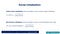Image link : http://bit.ly/2DTBOKV

a. Xavier Glorot uniform initialization of weights

Here the weights belong to a uniform distribution with in range of +x and -x, where x=(sqrt(6/(fan-in+fan-out)))

code for Xavier Glorot uniform initialization of weights

`model = Sequential()model.add(Dense(128, activation='relu', input_shape=(input_dim,), kernel_initializer='glorot_uniform'))model.add(Dense(64, activation='relu', kernel_initializer='glorot_uniform'))model.add(Dense(output_dim, activation='softmax'))`

Output for Xavier Glorot uniform initialization of weights

`Epoch 1/5 60000/60000 [==============================] - 4s 68us/step - loss: 0.3317 - acc: 0.9072 - val_loss: 0.1534 - val_acc: 0.9545 Epoch 2/5 60000/60000 [==============================] - 3s 55us/step - loss: 0.1303 - acc: 0.9614 - val_loss: 0.1124 - val_acc: 0.9679 Epoch 3/5 60000/60000 [==============================] - 3s 54us/step - loss: 0.0889 - acc: 0.9731 - val_loss: 0.0978 - val_acc: 0.9711 Epoch 4/5 60000/60000 [==============================] - 3s 54us/step - loss: 0.0668 - acc: 0.9795 - val_loss: 0.0863 - val_acc: 0.9735 Epoch 5/5 60000/60000 [==============================] - 3s 55us/step - loss: 0.0529 - acc: 0.9840 - val_loss: 0.0755 - val_acc: 0.9771`

Plot for outputs of Xavier Glorot uniform initialization of weights

Analysis of output

Here, with this Xavier Glorot uniform initialization, our model tends to perform very well. Although ,we can run it multiple times, our output won’t change.

b. Xavier Glorot normal initialization

Here the weights belongs to a normal distribution with mean=0 and variance= sqrt(2/(fan-in+fan-out)).

Code for Xavier Glorot normal initialization of weights

`model = Sequential()model.add(Dense(128, activation='relu', input_shape=(input_dim,), kernel_initializer='glorot_normal'))model.add(Dense(64, activation='relu', kernel_initializer='glorot_normal'))model.add(Dense(output_dim, activation='softmax'))`

Output for Xavier Glorot normal initialization of weights

`Epoch 1/5 60000/60000 [==============================] - 4s 66us/step - loss: 0.3296 - acc: 0.9064 - val_loss: 0.1628 - val_acc: 0.9492 Epoch 2/5 60000/60000 [==============================] - 3s 50us/step - loss: 0.1359 - acc: 0.9597 - val_loss: 0.1119 - val_acc: 0.9658 Epoch 3/5 60000/60000 [==============================] - 3s 51us/step - loss: 0.0945 - acc: 0.9721 - val_loss: 0.0929 - val_acc: 0.9706 Epoch 4/5 60000/60000 [==============================] - 3s 52us/step - loss: 0.0731 - acc: 0.9776 - val_loss: 0.0804 - val_acc: 0.9741 Epoch 5/5 60000/60000 [==============================] - 3s 51us/step - loss: 0.0576 - acc: 0.9824 - val_loss: 0.0707 - val_acc: 0.9783`

Plot for outputs of Xavier Glorot normal initialization of weights

Analysis of output

Here, with this Xavier Glorot normal initialization, our model also tends to perform very well. Although ,we can run it multiple times, our output won’t change .

The weights we set here are neither too big nor two small. Hence, we won’t face the problem of vanishing gradients and exploding gradients. Also, Xavier Glorot initialization helps in faster convergence to minima.

# . He initialization of weights

It is pronounced as hey initialization . This is also an advanced technique in initialization of weights. ReLU activation unit performs very well with this initialization .We consider only ,number of inputs in He- initialization .In He-initialization also, we have two types i.e., He-normal initialization and He-uniform initialization

a. He- uniform initialization of weights

Here the weights belongs to a uniform distribution within the range of +x and -x, where x=(sqrt(6/fan-in)).

Code for He- uniform initialization of weights

`model = Sequential()model.add(Dense(128, activation='relu', input_shape=(input_dim,), kernel_initializer='he_uniform'))model.add(Dense(64, activation='relu', kernel_initializer='he_uniform'))model.add(Dense(output_dim, activation='softmax'))`

Output for He-uniform initialization of weights

`Epoch 1/5 60000/60000 [==============================] - 4s 72us/step - loss: 0.3252 - acc: 0.9050 - val_loss: 0.1524 - val_acc: 0.9546 Epoch 2/5 60000/60000 [==============================] - 3s 52us/step - loss: 0.1314 - acc: 0.9611 - val_loss: 0.1104 - val_acc: 0.9671 Epoch 3/5 60000/60000 [==============================] - 3s 54us/step - loss: 0.0928 - acc: 0.9718 - val_loss: 0.0978 - val_acc: 0.9697 Epoch 4/5 60000/60000 [==============================] - 3s 53us/step - loss: 0.0703 - acc: 0.9786 - val_loss: 0.0890 - val_acc: 0.9740 Epoch 5/5 60000/60000 [==============================] - 3s 53us/step - loss: 0.0546 - acc: 0.9828 - val_loss: 0.0860 - val_acc: 0.9740`

Plot for He-uniform initialization of weights

Analysis of output

Here, in He-uniform initialization of weights we are only using the number of inputs. But, only with number of inputs, our model is performing quite descent with the He-uniform initialization of weights.

b. He- normal initialization of weights

Here the weights belongs to a normal distribution with mean=0 and variance= sqrt(2/(fan-in)).

Code for He- normal initialization of weights

`model = Sequential()model.add(Dense(128, activation='relu', input_shape=(input_dim,), kernel_initializer='he_normal'))model.add(Dense(64, activation='relu', kernel_initializer='he_normal'))model.add(Dense(output_dim, activation='softmax'))`

Output for He-normal initialization of weights

`Epoch 1/5 60000/60000 [==============================] - 4s 61us/step - loss: 0.3163 - acc: 0.9087 - val_loss: 0.1596 - val_acc: 0.9508 Epoch 2/5 60000/60000 [==============================] - 3s 45us/step - loss: 0.1319 - acc: 0.9610 - val_loss: 0.1163 - val_acc: 0.9625 Epoch 3/5 60000/60000 [==============================] - 3s 44us/step - loss: 0.0915 - acc: 0.9725 - val_loss: 0.0897 - val_acc: 0.9727 Epoch 4/5 60000/60000 [==============================] - 3s 45us/step - loss: 0.0693 - acc: 0.9795 - val_loss: 0.0878 - val_acc: 0.9735 Epoch 5/5 60000/60000 [==============================] - 3s 44us/step - loss: 0.0537 - acc: 0.9836 - val_loss: 0.0764 - val_acc: 0.9769`

Plot for outputs of He-normal initialization of weights

Analysis of output

Here, in He-normal initialization of weights we are only using the number of inputs. But, only with number of inputs, our model is performing well.

In He- initialization also,we set weights neither too big nor two small. Hence, we won’t face the problem of vanishing gradients and exploding gradients. Also, this initialization helps in faster convergence to minima.

How to choose right weight initialization ?

As their is no strong theory for choosing right weight initialization, we just have some rule of thumb methods i.e.,

• When we have sigmoid activation function, it is better to use Xavier Glorot initialization of weights.
• When we have ReLU activation function, it is better to use He-initialization of weights.

Mostly, Convolutional neural network will use ReLU activation function and it use’s he-initialization.

References

Hope this article helps you.

Special thanks to team at appliedai for helping me and motivating me .Image link : http://bit.ly/2DcxaX0

Thank you for reading..!

Welcome to a place where words matter. On Medium, smart voices and original ideas take center stage - with no ads in sight. Watch
Follow all the topics you care about, and we’ll deliver the best stories for you to your homepage and inbox. Explore
Get unlimited access to the best stories on Medium — and support writers while you’re at it. Just \$5/month. Upgrade